Data Bootcamp: Demography¶

David Backus

We love demography, specifically the dynamics of population growth and decline. You can drill down seemingly without end, as this terrific graphic about causes of death suggests.

We take a look here at the UN's population data: the age distribution of the population, life expectancy, fertility (the word we use for births), and mortality (deaths). Explore the website, it's filled with interesting data. There are other sources that cover longer time periods, and for some countries you can get detailed data on specific things (causes of death, for example).

We use a number of countries as examples, but Japan and China are the most striking. The code is written so that the country is easily changed.

This IPython notebook was created by Dave Backus, Chase Coleman, and Spencer Lyon for the NYU Stern course Data Bootcamp.

Preliminaries¶

Import statements and a date check for future reference.

In :
# import packages
import pandas as pd                   # data management
import matplotlib.pyplot as plt       # graphics
import matplotlib as mpl              # graphics parameters
import numpy as np                    # numerical calculations

# IPython command, puts plots in notebook
%matplotlib inline

# check Python version
import datetime as dt
import sys
print('Today is', dt.date.today())
print('What version of Python are we running? \n', sys.version, sep='')
Today is 2016-03-23
What version of Python are we running?
3.5.1 |Anaconda 2.5.0 (64-bit)| (default, Jan 29 2016, 15:01:46) [MSC v.1900 64 bit (AMD64)]
In [ ]:

Population by age¶

We have both "estimates" of the past (1950-2015) and "projections" of the future (out to 2100). Here we focus on the latter, specifically what the UN refers to as the medium variant: their middle of the road projection. It gives us a sense of how Japan's population might change over the next century.

It takes a few seconds to read the data.

What are the numbers? Thousands of people in various 5-year age categories.

In :
url1 = 'http://esa.un.org/unpd/wpp/DVD/Files/'
url2 = '1_Indicators%20(Standard)/EXCEL_FILES/1_Population/'
url3 = 'WPP2015_POP_F07_1_POPULATION_BY_AGE_BOTH_SEXES.XLS'
url = url1 + url2 + url3

cols = [2, 5] + list(range(6,28))
#est = pd.read_excel(url, sheetname=0, skiprows=16, parse_cols=cols, na_values=['…'])
prj = pd.read_excel(url, sheetname=1, skiprows=16, parse_cols=cols, na_values=['…'])

Out:
Major area, region, country or area * Reference date (as of 1 July) 0-4 5-9 10-14 15-19
0 WORLD 2015 670928.185 637448.895 607431.299 590069.337
1 WORLD 2020 677599.590 664282.610 634568.409 604322.921
2 WORLD 2025 673174.914 671929.973 661684.410 631509.113
In :
# rename some variables
pop = prj
names = list(pop)
pop = pop.rename(columns={names: 'Country',
names: 'Year'})
# select country and years
country = ['Japan']
years     = [2015, 2055, 2095]
pop = pop[pop['Country'].isin(country) & pop['Year'].isin(years)]
pop = pop.drop(['Country'], axis=1)

# set index = Year
# divide by 1000 to convert numbers from thousands to millions
pop = pop.set_index('Year')/1000

Out:
0-4 5-9 10-14 15-19 20-24 25-29 30-34 35-39
Year
2015 5.269038 5.398973 5.603638 5.960784 6.111768 6.843421 7.455687 8.345753
2055 4.271907 4.371016 4.458145 4.557117 4.685414 4.829637 5.013420 5.236482
2095 3.720541 3.789596 3.860493 3.938189 4.025477 4.106967 4.194272 4.290983
In :
# transpose (T) so that index = age
pop = pop.T
Out:
Year 2015 2055 2095
0-4 5.269038 4.271907 3.720541
5-9 5.398973 4.371016 3.789596
10-14 5.603638 4.458145 3.860493
In :
ax = pop.plot(kind='bar',
color='blue',
alpha=0.5, subplots=True, sharey=True, figsize=(8,6))

for axnum in range(len(ax)):
ax[axnum].set_title('')
ax[axnum].set_ylabel('Millions')

ax.set_title('Population by age', fontsize=14, loc='left')
Out:
<matplotlib.text.Text at 0xa259e10>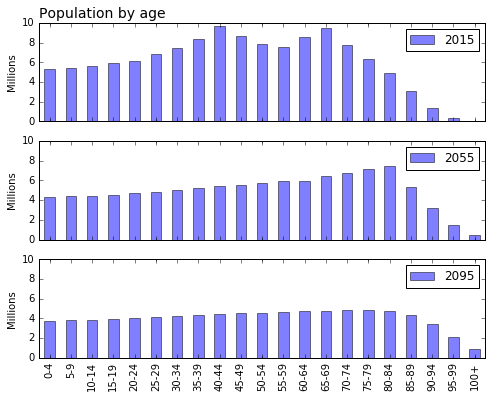In [ ]:

Exercise. What do you see here? What else would you like to know?

Exercise. Adapt the preceeding code to do the same thing for China. Or some other country that sparks your interest.

In [ ]:

Fertility: aka birth rates¶

We might wonder, why is the population falling in Japan? Other countries? Well, one reason is that birth rates are falling. Demographers call this fertility. Here we look at the fertility using the same UN source as the previous example. We look at two variables: total fertility and fertility by age of mother. In both cases we explore the numbers to date, but the same files contain projections of future fertility.

In :
# fertility overall
uft  = 'http://esa.un.org/unpd/wpp/DVD/Files/'
uft += '1_Indicators%20(Standard)/EXCEL_FILES/'
uft += '2_Fertility/WPP2015_FERT_F04_TOTAL_FERTILITY.XLS'

cols =  + list(range(5,18))
ftot = pd.read_excel(uft, sheetname=0, skiprows=16, parse_cols=cols, na_values=['…'])

Out:
Major area, region, country or area * 1950-1955 1955-1960 1960-1965 1965-1970 1970-1975
0 WORLD 4.961571 4.898665 5.024379 4.922202 4.475478
1 More developed regions 2.823786 2.807368 2.685900 2.387534 2.150816
2 Less developed regions 6.075417 5.941033 6.129418 6.034843 5.416602
In :
# rename some variables
names = list(ftot)
f = ftot.rename(columns={names: 'Country'})

# select countries
countries = ['China', 'Japan', 'Germany', 'United States of America']
f = f[f['Country'].isin(countries)]

# shape
f = f.set_index('Country').T
f = f.rename(columns={'United States of America': 'United States'})
f.tail(3)
Out:
Country China Japan Germany United States
2000-2005 1.50 1.2980 1.3513 2.0420
2005-2010 1.53 1.3388 1.3623 2.0590
2010-2015 1.55 1.3960 1.3909 1.8902
In :
fig, ax = plt.subplots()
f.plot(ax=ax, kind='line', alpha=0.5, lw=3, figsize=(6.5, 4))
ax.set_title('Fertility (births per woman, lifetime)', fontsize=14, loc='left')
ax.legend(loc='best', fontsize=10, handlelength=2, labelspacing=0.15)
ax.set_ylim(ymin=0)
ax.hlines(2.1, -1, 13, linestyles='dashed')
ax.text(8.5, 2.4, 'Replacement = 2.1')
Out:
<matplotlib.text.Text at 0x92c4278>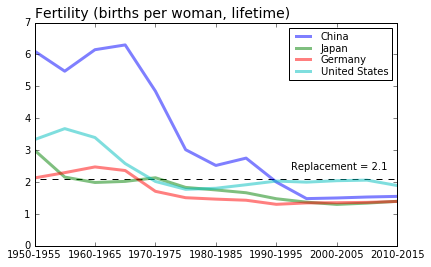Exercise. What do you see here? What else would you like to know?

Exercise. Add Canada to the figure. How does it compare to the others? What other countries would you be interested in?

In [ ]:

Life expectancy¶

One of the bottom line summary numbers for mortality is life expectancy: if mortaility rates fall, people live longer, on average. Here we look at life expectancy at birth. There are also numbers for life expectancy given than you live to some specific age; for example, life expectancy given that you survive to age 60.

In :
# life expectancy at birth, both sexes
ule  = 'http://esa.un.org/unpd/wpp/DVD/Files/1_Indicators%20(Standard)/EXCEL_FILES/3_Mortality/'
ule += 'WPP2015_MORT_F07_1_LIFE_EXPECTANCY_0_BOTH_SEXES.XLS'

cols =  + list(range(5,34))
le  = pd.read_excel(ule, sheetname=0, skiprows=16, parse_cols=cols, na_values=['…'])

Out:
Major area, region, country or area * 1950-1955 1955-1960 1960-1965 1965-1970 1970-1975 1975-1980 1980-1985 1985-1990 1990-1995
0 WORLD 46.807955 49.211594 51.069722 55.378481 58.049389 60.212254 61.989053 63.610776 64.536781
1 More developed regions 64.669835 67.679537 69.440381 70.281907 71.063794 71.960446 72.806852 73.901457 74.107521
2 Less developed regions 41.508118 43.882493 46.017378 51.441078 54.770858 57.342650 59.418113 61.229860 62.453399
In :
# rename some variables
oldname = list(le)
l = le.rename(columns={oldname: 'Country'})
Out:
Country 1950-1955 1955-1960 1960-1965 1965-1970 1970-1975 1975-1980 1980-1985
0 WORLD 46.807955 49.211594 51.069722 55.378481 58.049389 60.212254 61.989053
1 More developed regions 64.669835 67.679537 69.440381 70.281907 71.063794 71.960446 72.806852
2 Less developed regions 41.508118 43.882493 46.017378 51.441078 54.770858 57.342650 59.418113
In :
# select countries
countries = ['China', 'Japan', 'Germany', 'United States of America']
l = l[l['Country'].isin(countries)]

# shape
l = l.set_index('Country').T
l = l.rename(columns={'United States of America': 'United States'})
l.tail()
Out:
Country China Japan Germany United States
1990-1995 69.386 79.447 75.878 75.617
1995-2000 70.587 80.475 77.212 76.404
2000-2005 72.852 81.829 78.573 77.133
2005-2010 74.438 82.621 79.757 78.113
2010-2015 75.432 83.298 80.647 78.873
In :
fig, ax = plt.subplots()
l.plot(ax=ax, kind='line', alpha=0.5, lw=3, figsize=(6, 8), grid=True)
ax.set_title('Life expectancy at birth', fontsize=14, loc='left')
ax.set_ylabel('Life expectancy in years')
ax.legend(loc='best', fontsize=10, handlelength=2, labelspacing=0.15)
ax.set_ylim(ymin=0)
Out:
(0, 85.0)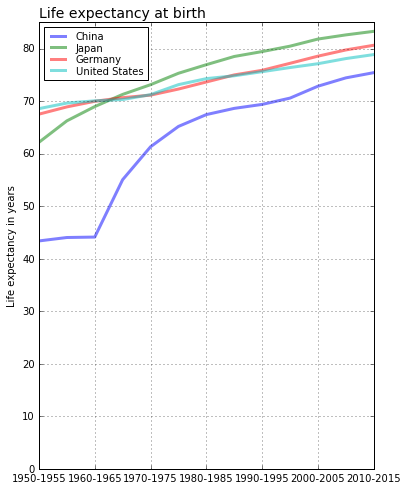Exercise. What other countries would you like to see? Can you add them? The code below generates a list.

In :
countries = le.rename(columns={oldname: 'Country'})['Country']

Exercise. Why do you think the US is falling behind? What would you look at to verify your conjecture?

In [ ]:

Mortality: aka death rates¶

Another thing that affects the age distribution of the population is the mortality rate: if mortality rates fall people live longer, on average. Here we look at how mortality rates have changed over the past 60+ years. Roughly speaking, people live an extra five years every generation. Which is a lot. Some of you will live to be a hundred. (Look at the 100+ agen category over time for Japan.)

The experts look at mortality rates by age. The UN has a whole page devoted to mortality numbers. We take 5-year mortality rates from the Abridged Life Table.

The numbers are percentages of people in a given age group who die over a 5-year period. 0.1 means that 90 percent of an age group is still alive in five years.

In :
# mortality overall
url  = 'http://esa.un.org/unpd/wpp/DVD/Files/'
url += '1_Indicators%20(Standard)/EXCEL_FILES/3_Mortality/'
url += 'WPP2015_MORT_F17_1_ABRIDGED_LIFE_TABLE_BOTH_SEXES.XLS'

cols = [2, 5, 6, 7, 9]
mort = pd.read_excel(url, sheetname=0, skiprows=16, parse_cols=cols, na_values=['…'])
mort.tail(3)
Out:
Major area, region, country or area * Period Age (x) Age interval (n) Probability of dying q(x,n)
59524 Tonga 2010-2015 75 5 0.279867
59525 Tonga 2010-2015 80 5 0.397735
59526 Tonga 2010-2015 85 15 NaN
In :
# change names
names = list(mort)
m = mort.rename(columns={names: 'Country', names: 'Age', names: 'Interval', names: 'Mortality'})
Out:
Country Period Age Interval Mortality
0 WORLD 1950-1955 0 1 0.141804
1 WORLD 1950-1955 1 4 0.085487
2 WORLD 1950-1955 5 5 0.031513

Comment. At this point, we need to pivot the data. That's not something we've done before, so take it as simply something we can do easily if we have to. We're going to do this twice to produce different graphs:

• Compare countries for the same period.
• Compare different periods for the same country.
In :
# compare countries for most recent period
countries = ['China', 'Japan', 'Germany', 'United States of America']
mt = m[m['Country'].isin(countries) & m['Interval'].isin() & m['Period'].isin(['2010-2015'])]
print('Dimensions:', mt.shape)

mp = mt.pivot(index='Age', columns='Country', values='Mortality')
Dimensions: (64, 5)
Out:
Country China Germany Japan United States of America
Age
5 0.001756 0.000397 0.000462 0.000597
10 0.001286 0.000456 0.000437 0.000714
15 0.001844 0.001300 0.001141 0.002264
In :
fig, ax = plt.subplots()
mp.plot(ax=ax, kind='line', alpha=0.5, linewidth=3,
#        logy=True,
figsize=(6, 4))
ax.set_title('Mortality by age', fontsize=14, loc='left')
ax.set_ylabel('Mortality Rate (log scale)')
ax.legend(loc='best', fontsize=10, handlelength=2, labelspacing=0.15)
Out:
<matplotlib.legend.Legend at 0x9191390>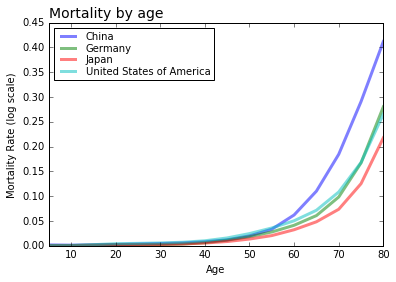Exercises.

• What country's old people have the lowest mortality?
• What do you see here for the US? Why is our life expectancy shorter?
• What other countries would you like to see? Can you adapt the code to show them?
• Anything else cross your mind?
In [ ]:

In :
# compare periods for the one country -- countries is China
mt = m[m['Country'].isin([countries]) & m['Interval'].isin()]
print('Dimensions:', mt.shape)

mp = mt.pivot(index='Age', columns='Period', values='Mortality')
mp = mp[[0, 6, 12]]
Dimensions: (208, 5)
Out:
Period 1950-1955 1980-1985 2010-2015
Age
5 0.043088 0.006487 0.001756
10 0.025641 0.003300 0.001286
15 0.026845 0.004172 0.001844
In :
fig, ax = plt.subplots()
mp.plot(ax=ax, kind='line', alpha=0.5, linewidth=3,
#        logy=True,
figsize=(6, 4))
ax.set_title('Mortality over time', fontsize=14, loc='left')
ax.set_ylabel('Mortality Rate (log scale)')
ax.legend(loc='best', fontsize=10, handlelength=2, labelspacing=0.15)
Out:
<matplotlib.legend.Legend at 0xd7d7dd8>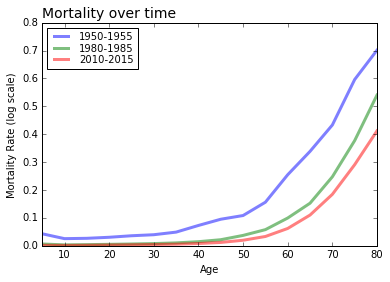Exercise. What do you see? What else would you like to know?

Exercise. Repeat this graph for the United States? How does it compare?

In [ ]:

In [ ]: